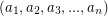# Primitive pythagorean n-tuple tree

 Importance: Medium ✭✭
 Author(s):
 Subject: Number Theory
 Keywords:
 Posted by: tsihonglau on: April 24th, 2011
Conjecture   Find linear transformation construction of primitive pythagorean n-tuple tree!

Primitive pythagorean n-tuple is a n-tuplesuch thatand the greatest common divisor ofis 1.

There are at least two known linear transformation construction of primitive pythagorean triple tree!

Wikipedia

Is there any other linear transformation construction of primitive pythagorean triple tree?

Moreover, find linear transformation construction of primitive pythagorean n-tuple tree!

## Bibliography

* indicates original appearance(s) of problem.

### Linear transformation of primitive pythagorean n-tuple

Is it true that for primitive pythagorean n-tuple (a_1, a_2...... a_n), if there are (n-2) even terms and 1 odd term in the summation side of the equation and a_n is odd, a linear transformation can be made? And can we find any such primitive pythagorean n-tuple where a_n is even?